# 10.2 The hyperbola  (Page 9/13)

 Page 9 / 13

$\frac{{\left(y-6\right)}^{2}}{36}-\frac{{\left(x+1\right)}^{2}}{16}=1$

$\frac{{\left(x-2\right)}^{2}}{49}-\frac{{\left(y+7\right)}^{2}}{49}=1$

$\frac{{\left(x-2\right)}^{2}}{{7}^{2}}-\frac{{\left(y+7\right)}^{2}}{{7}^{2}}=1;\text{\hspace{0.17em}}$ vertices: $\text{\hspace{0.17em}}\left(9,-7\right),\left(-5,-7\right);\text{\hspace{0.17em}}$ foci: $\text{\hspace{0.17em}}\left(2+7\sqrt{2},-7\right),\left(2-7\sqrt{2},-7\right);\text{\hspace{0.17em}}$ asymptotes: $\text{\hspace{0.17em}}y=x-9,y=-x-5$

$4{x}^{2}-8x-9{y}^{2}-72y+112=0$

$-9{x}^{2}-54x+9{y}^{2}-54y+81=0$

$\frac{{\left(x+3\right)}^{2}}{{3}^{2}}-\frac{{\left(y-3\right)}^{2}}{{3}^{2}}=1;\text{\hspace{0.17em}}$ vertices: $\text{\hspace{0.17em}}\left(0,3\right),\left(-6,3\right);\text{\hspace{0.17em}}$ foci: $\text{\hspace{0.17em}}\left(-3+3\sqrt{2},1\right),\left(-3-3\sqrt{2},1\right);\text{\hspace{0.17em}}$ asymptotes: $\text{\hspace{0.17em}}y=x+6,y=-x$

$4{x}^{2}-24x-36{y}^{2}-360y+864=0$

$-4{x}^{2}+24x+16{y}^{2}-128y+156=0$

$\frac{{\left(y-4\right)}^{2}}{{2}^{2}}-\frac{{\left(x-3\right)}^{2}}{{4}^{2}}=1;\text{\hspace{0.17em}}$ vertices: $\text{\hspace{0.17em}}\left(3,6\right),\left(3,2\right);\text{\hspace{0.17em}}$ foci: $\text{\hspace{0.17em}}\left(3,4+2\sqrt{5}\right),\left(3,4-2\sqrt{5}\right);\text{\hspace{0.17em}}$ asymptotes: $\text{\hspace{0.17em}}y=\frac{1}{2}\left(x-3\right)+4,y=-\frac{1}{2}\left(x-3\right)+4$

$-4{x}^{2}+40x+25{y}^{2}-100y+100=0$

${x}^{2}+2x-100{y}^{2}-1000y+2401=0$

$\frac{{\left(y+5\right)}^{2}}{{7}^{2}}-\frac{{\left(x+1\right)}^{2}}{{70}^{2}}=1;\text{\hspace{0.17em}}$ vertices: $\text{\hspace{0.17em}}\left(-1,2\right),\left(-1,-12\right);\text{\hspace{0.17em}}$ foci: $\text{\hspace{0.17em}}\left(-1,-5+7\sqrt{101}\right),\left(-1,-5-7\sqrt{101}\right);\text{\hspace{0.17em}}$ asymptotes: $\text{\hspace{0.17em}}y=\frac{1}{10}\left(x+1\right)-5,y=-\frac{1}{10}\left(x+1\right)-5$

$-9{x}^{2}+72x+16{y}^{2}+16y+4=0$

$4{x}^{2}+24x-25{y}^{2}+200y-464=0$

$\frac{{\left(x+3\right)}^{2}}{{5}^{2}}-\frac{{\left(y-4\right)}^{2}}{{2}^{2}}=1;\text{\hspace{0.17em}}$ vertices: $\text{\hspace{0.17em}}\left(2,4\right),\left(-8,4\right);\text{\hspace{0.17em}}$ foci: $\text{\hspace{0.17em}}\left(-3+\sqrt{29},4\right),\left(-3-\sqrt{29},4\right);\text{\hspace{0.17em}}$ asymptotes: $\text{\hspace{0.17em}}y=\frac{2}{5}\left(x+3\right)+4,y=-\frac{2}{5}\left(x+3\right)+4$

For the following exercises, find the equations of the asymptotes for each hyperbola.

$\frac{{y}^{2}}{{3}^{2}}-\frac{{x}^{2}}{{3}^{2}}=1$

$\frac{{\left(x-3\right)}^{2}}{{5}^{2}}-\frac{{\left(y+4\right)}^{2}}{{2}^{2}}=1$

$y=\frac{2}{5}\left(x-3\right)-4,y=-\frac{2}{5}\left(x-3\right)-4$

$\frac{{\left(y-3\right)}^{2}}{{3}^{2}}-\frac{{\left(x+5\right)}^{2}}{{6}^{2}}=1$

$9{x}^{2}-18x-16{y}^{2}+32y-151=0$

$y=\frac{3}{4}\left(x-1\right)+1,y=-\frac{3}{4}\left(x-1\right)+1$

$16{y}^{2}+96y-4{x}^{2}+16x+112=0$

## Graphical

For the following exercises, sketch a graph of the hyperbola, labeling vertices and foci.

$\frac{{x}^{2}}{49}-\frac{{y}^{2}}{16}=1$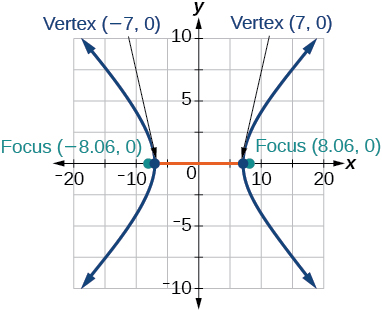$\frac{{x}^{2}}{64}-\frac{{y}^{2}}{4}=1$

$\frac{{y}^{2}}{9}-\frac{{x}^{2}}{25}=1$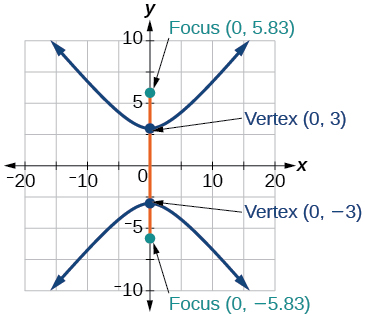$81{x}^{2}-9{y}^{2}=1$

$\frac{{\left(y+5\right)}^{2}}{9}-\frac{{\left(x-4\right)}^{2}}{25}=1$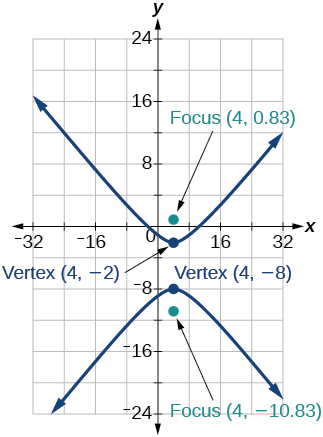$\frac{{\left(x-2\right)}^{2}}{8}-\frac{{\left(y+3\right)}^{2}}{27}=1$

$\frac{{\left(y-3\right)}^{2}}{9}-\frac{{\left(x-3\right)}^{2}}{9}=1$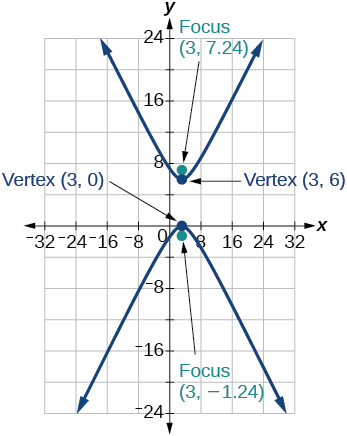$-4{x}^{2}-8x+16{y}^{2}-32y-52=0$

${x}^{2}-8x-25{y}^{2}-100y-109=0$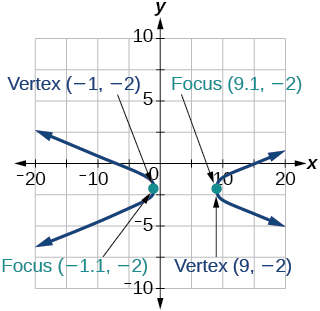$-{x}^{2}+8x+4{y}^{2}-40y+88=0$

$64{x}^{2}+128x-9{y}^{2}-72y-656=0$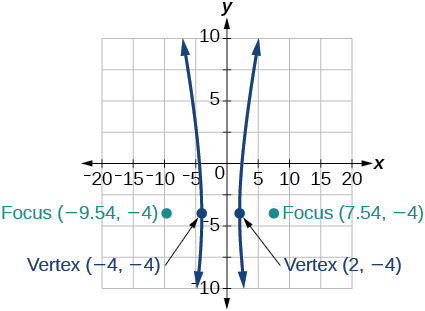$16{x}^{2}+64x-4{y}^{2}-8y-4=0$

$-100{x}^{2}+1000x+{y}^{2}-10y-2575=0$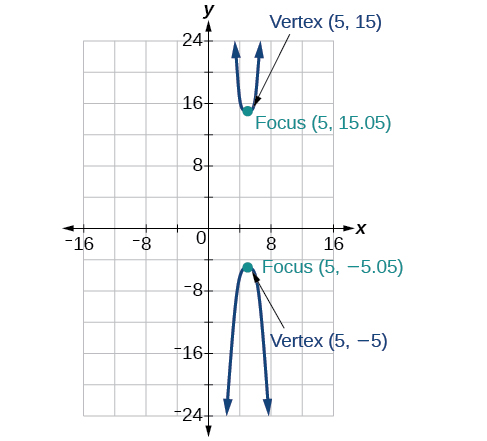$4{x}^{2}+16x-4{y}^{2}+16y+16=0$

For the following exercises, given information about the graph of the hyperbola, find its equation.

Vertices at $\text{\hspace{0.17em}}\left(3,0\right)\text{\hspace{0.17em}}$ and $\text{\hspace{0.17em}}\left(-3,0\right)\text{\hspace{0.17em}}$ and one focus at $\text{\hspace{0.17em}}\left(5,0\right).$

$\frac{{x}^{2}}{9}-\frac{{y}^{2}}{16}=1$

Vertices at $\text{\hspace{0.17em}}\left(0,6\right)\text{\hspace{0.17em}}$ and $\text{\hspace{0.17em}}\left(0,-6\right)\text{\hspace{0.17em}}$ and one focus at $\text{\hspace{0.17em}}\left(0,-8\right).$

Vertices at $\text{\hspace{0.17em}}\left(1,1\right)\text{\hspace{0.17em}}$ and $\text{\hspace{0.17em}}\left(11,1\right)\text{\hspace{0.17em}}$ and one focus at $\text{\hspace{0.17em}}\left(12,1\right).$

$\frac{{\left(x-6\right)}^{2}}{25}-\frac{{\left(y-1\right)}^{2}}{11}=1$

Center: $\text{\hspace{0.17em}}\left(0,0\right);$ vertex: $\text{\hspace{0.17em}}\left(0,-13\right);$ one focus: $\text{\hspace{0.17em}}\left(0,\sqrt{313}\right).$

Center: $\text{\hspace{0.17em}}\left(4,2\right);$ vertex: $\text{\hspace{0.17em}}\left(9,2\right);$ one focus: $\text{\hspace{0.17em}}\left(4+\sqrt{26},2\right).$

$\frac{{\left(x-4\right)}^{2}}{25}-\frac{{\left(y-2\right)}^{2}}{1}=1$

Center: $\text{\hspace{0.17em}}\left(3,5\right);\text{\hspace{0.17em}}$ vertex: $\text{\hspace{0.17em}}\left(3,11\right);\text{\hspace{0.17em}}$ one focus: $\text{\hspace{0.17em}}\left(3,5+2\sqrt{10}\right).$

For the following exercises, given the graph of the hyperbola, find its equation.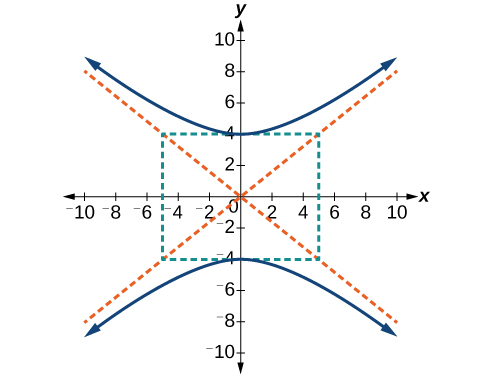$\frac{{y}^{2}}{16}-\frac{{x}^{2}}{25}=1$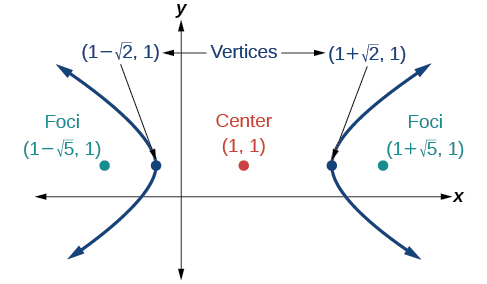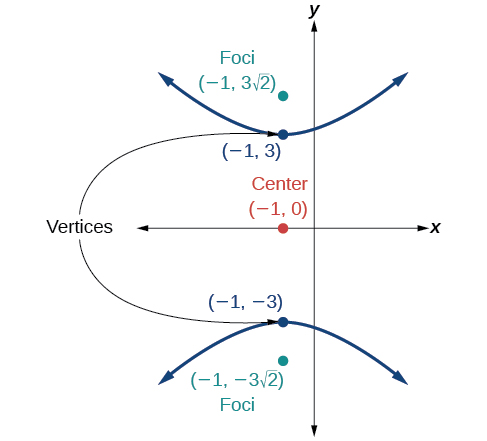$\frac{{y}^{2}}{9}-\frac{{\left(x+1\right)}^{2}}{9}=1$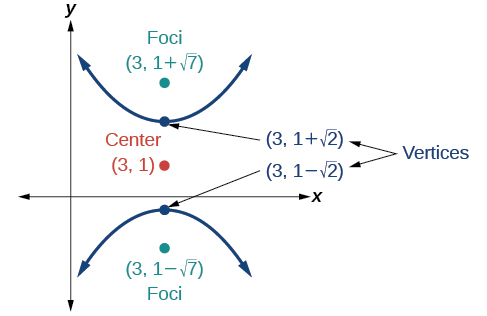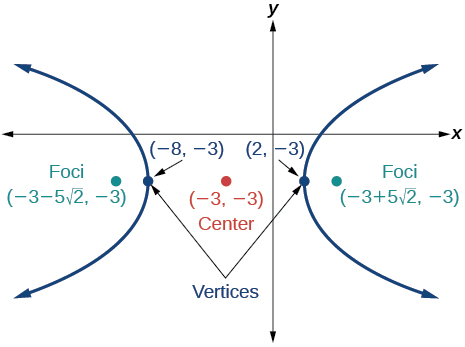$\frac{{\left(x+3\right)}^{2}}{25}-\frac{{\left(y+3\right)}^{2}}{25}=1$

## Extensions

For the following exercises, express the equation for the hyperbola as two functions, with $\text{\hspace{0.17em}}y\text{\hspace{0.17em}}$ as a function of $\text{\hspace{0.17em}}x.\text{\hspace{0.17em}}$ Express as simply as possible. Use a graphing calculator to sketch the graph of the two functions on the same axes.

$\frac{{x}^{2}}{4}-\frac{{y}^{2}}{9}=1$

$\frac{{y}^{2}}{9}-\frac{{x}^{2}}{1}=1$

$y\left(x\right)=3\sqrt{{x}^{2}+1},y\left(x\right)=-3\sqrt{{x}^{2}+1}$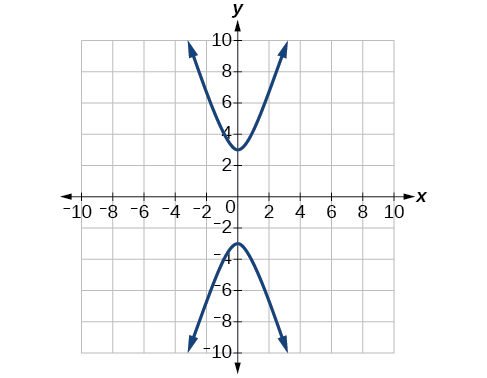$\frac{{\left(x-2\right)}^{2}}{16}-\frac{{\left(y+3\right)}^{2}}{25}=1$

$-4{x}^{2}-16x+{y}^{2}-2y-19=0$

$y\left(x\right)=1+2\sqrt{{x}^{2}+4x+5},y\left(x\right)=1-2\sqrt{{x}^{2}+4x+5}$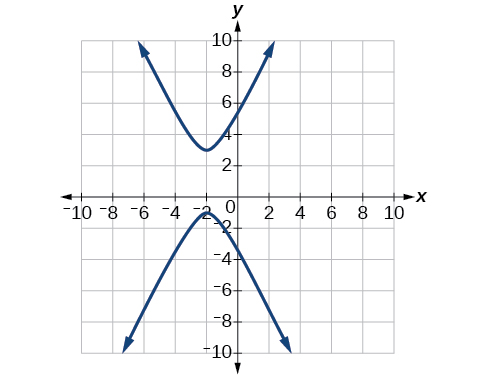$4{x}^{2}-24x-{y}^{2}-4y+16=0$

## Real-world applications

For the following exercises, a hedge is to be constructed in the shape of a hyperbola near a fountain at the center of the yard. Find the equation of the hyperbola and sketch the graph.

The hedge will follow the asymptotes and its closest distance to the center fountain is 5 yards.

$\frac{{x}^{2}}{25}-\frac{{y}^{2}}{25}=1$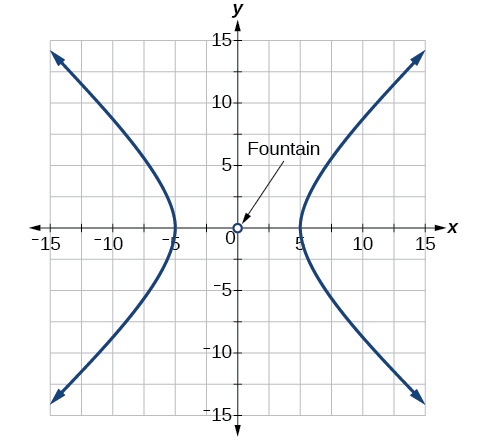The hedge will follow the asymptotes and its closest distance to the center fountain is 6 yards.

The hedge will follow the asymptotes $\text{\hspace{0.17em}}y=\frac{1}{2}x\text{\hspace{0.17em}}$ and $\text{\hspace{0.17em}}y=-\frac{1}{2}x,$ and its closest distance to the center fountain is 10 yards.

$\frac{{x}^{2}}{100}-\frac{{y}^{2}}{25}=1$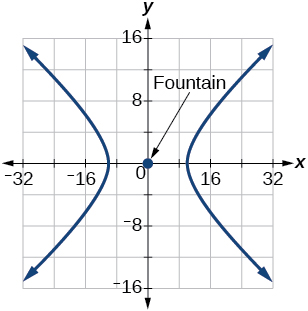The hedge will follow the asymptotes $\text{\hspace{0.17em}}y=\frac{2}{3}x\text{\hspace{0.17em}}$ and $\text{\hspace{0.17em}}y=-\frac{2}{3}x,$ and its closest distance to the center fountain is 12 yards.

The hedge will follow the asymptotes and its closest distance to the center fountain is 20 yards.

$\frac{{x}^{2}}{400}-\frac{{y}^{2}}{225}=1$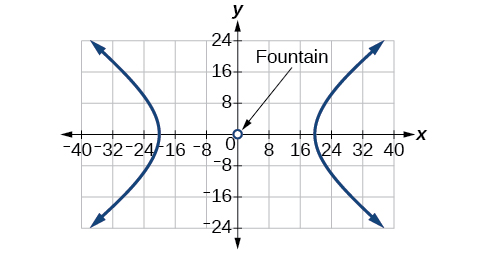For the following exercises, assume an object enters our solar system and we want to graph its path on a coordinate system with the sun at the origin and the x-axis as the axis of symmetry for the object's path. Give the equation of the flight path of each object using the given information.

The object enters along a path approximated by the line $\text{\hspace{0.17em}}y=x-2\text{\hspace{0.17em}}$ and passes within 1 au (astronomical unit) of the sun at its closest approach, so that the sun is one focus of the hyperbola. It then departs the solar system along a path approximated by the line $\text{\hspace{0.17em}}y=-x+2.\text{\hspace{0.17em}}$

The object enters along a path approximated by the line $\text{\hspace{0.17em}}y=2x-2\text{\hspace{0.17em}}$ and passes within 0.5 au of the sun at its closest approach, so the sun is one focus of the hyperbola. It then departs the solar system along a path approximated by the line $\text{\hspace{0.17em}}y=-2x+2.\text{\hspace{0.17em}}$

$\frac{{\left(x-1\right)}^{2}}{0.25}-\frac{{y}^{2}}{0.75}=1$

The object enters along a path approximated by the line $\text{\hspace{0.17em}}y=0.5x+2\text{\hspace{0.17em}}$ and passes within 1 au of the sun at its closest approach, so the sun is one focus of the hyperbola. It then departs the solar system along a path approximated by the line $\text{\hspace{0.17em}}y=-0.5x-2.\text{\hspace{0.17em}}$

The object enters along a path approximated by the line $\text{\hspace{0.17em}}y=\frac{1}{3}x-1\text{\hspace{0.17em}}$ and passes within 1 au of the sun at its closest approach, so the sun is one focus of the hyperbola. It then departs the solar system along a path approximated by the line

$\frac{{\left(x-3\right)}^{2}}{4}-\frac{{y}^{2}}{5}=1$

The object It enters along a path approximated by the line $\text{\hspace{0.17em}}y=3x-9\text{\hspace{0.17em}}$ and passes within 1 au of the sun at its closest approach, so the sun is one focus of the hyperbola. It then departs the solar system along a path approximated by the line $\text{\hspace{0.17em}}y=-3x+9.\text{\hspace{0.17em}}$

a colony of bacteria is growing exponentially doubling in size every 100 minutes. how much minutes will it take for the colony of bacteria to triple in size
what is the importance knowing the graph of circular functions?
can get some help basic precalculus
What do you need help with?
Andrew
how to convert general to standard form with not perfect trinomial
can get some help inverse function
ismail
Rectangle coordinate
how to find for x
it depends on the equation
Robert
whats a domain
The domain of a function is the set of all input on which the function is defined. For example all real numbers are the Domain of any Polynomial function.
Spiro
foci (–7,–17) and (–7,17), the absolute value of the differenceof the distances of any point from the foci is 24.
difference between calculus and pre calculus?
give me an example of a problem so that I can practice answering
x³+y³+z³=42
Robert
dont forget the cube in each variable ;)
Robert
of she solves that, well ... then she has a lot of computational force under her command ....
Walter
what is a function?
I want to learn about the law of exponent
explain this
what is functions?
A mathematical relation such that every input has only one out.
Spiro
yes..it is a relationo of orders pairs of sets one or more input that leads to a exactly one output.
Mubita
Is a rule that assigns to each element X in a set A exactly one element, called F(x), in a set B.
RichieRich
If the plane intersects the cone (either above or below) horizontally, what figure will be created?

#### Get Jobilize Job Search Mobile App in your pocket Now!ByByBy Nicole BartelsBy Abishek DevarajByBy OpenStaxBy John GabrieliBy Inderjeet BrarBy OpenStaxBy Cath YuByBy OpenStax